# Chapter 3 Solving Inequalities Inequality Signs An inequality

• Slides: 39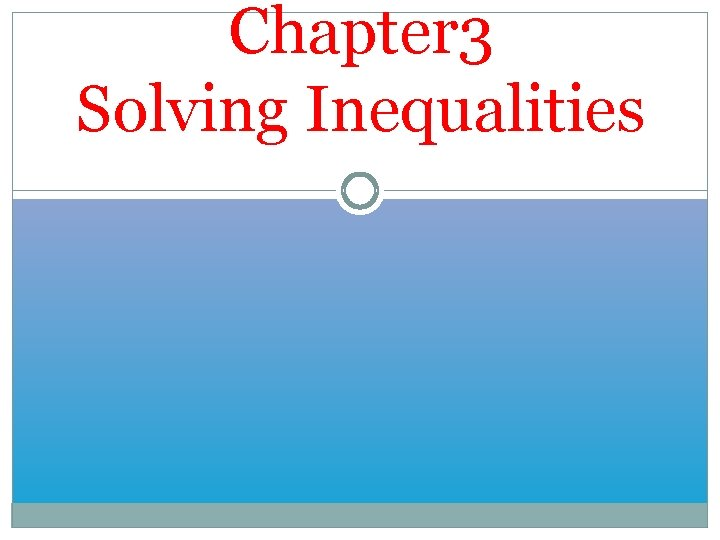Chapter 3 Solving InequalitiesInequality Signs An inequality is like an equation, but instead of an equal sign (=) it has one of these signs: < : less than ≤ : less than or equal to > : greater than ≥ : greater than or equal to“x < 5” means that whatever value x has, it must be less than 5. What could x be?“x ≥ -2” means that whatever value x has, it must be greater than or equal to -2. What could x be?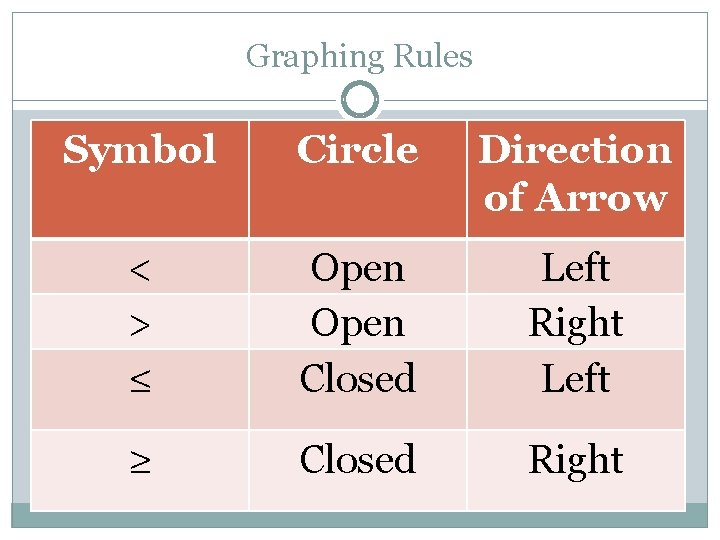Graphing Rules Symbol Circle Direction of Arrow < > ≤ Open Closed Left Right Left ≥ Closed RightExamples: x < 5 x > -2 x ≤ -8 x ≥ 4You Try: x < -6 x > 2 x ≤ 0 x ≥ -7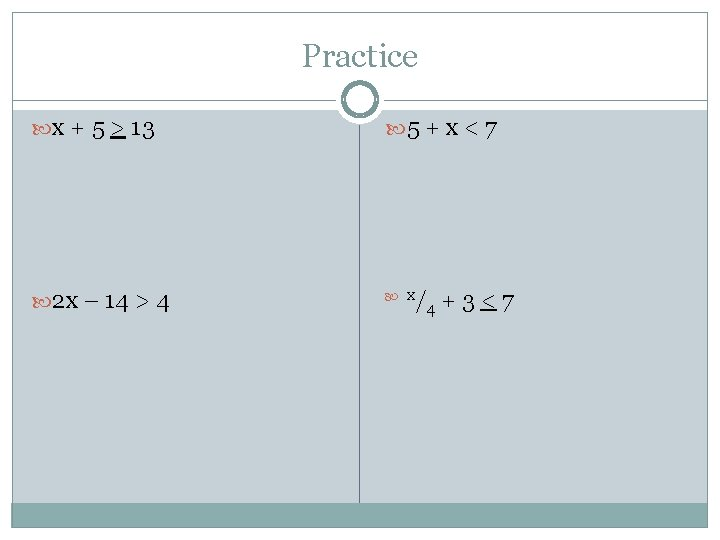Practice x + 5 > 13 5 + x < 7 2 x – 14 > 4 x /4 + 3 < 7DAY 2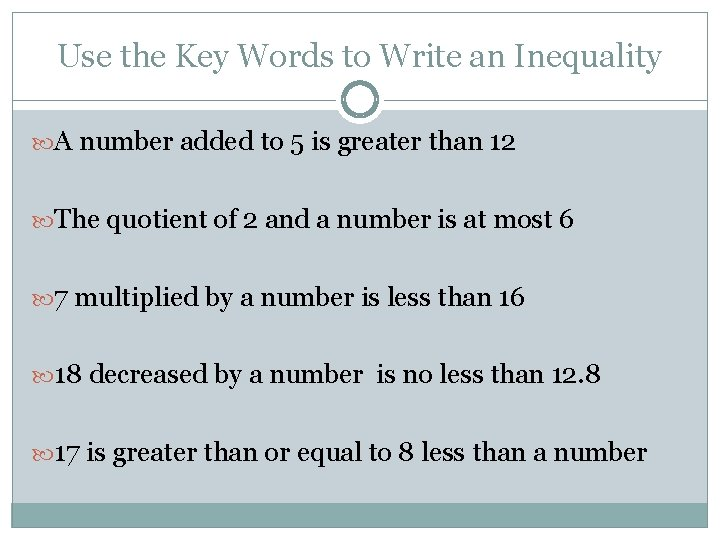Use the Key Words to Write an Inequality A number added to 5 is greater than 12 The quotient of 2 and a number is at most 6 7 multiplied by a number is less than 16 18 decreased by a number is no less than 12. 8 17 is greater than or equal to 8 less than a numberSolving One-Step Inequalities Addition Property for Inequalities X-15<73Solving One-Step Inequalities y+15<25Solving One-Step Inequalities Multiply both sides by the reciprocal of the coefficient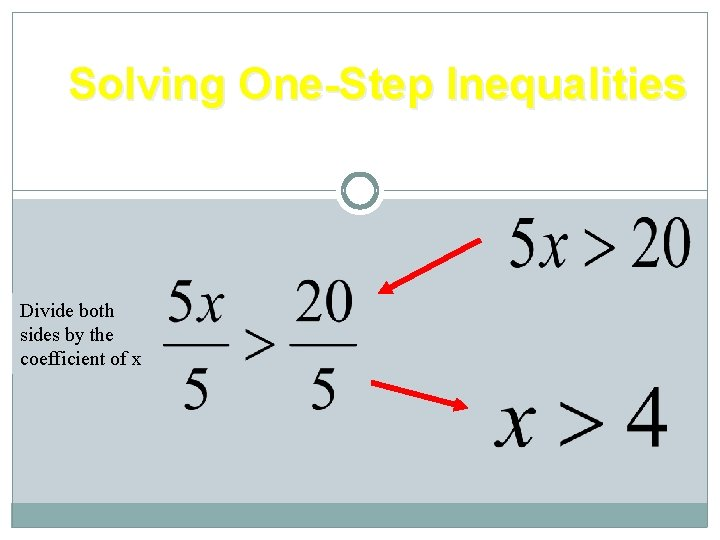Solving One-Step Inequalities Division Property for Inequalities Divide both sides by the coefficient of x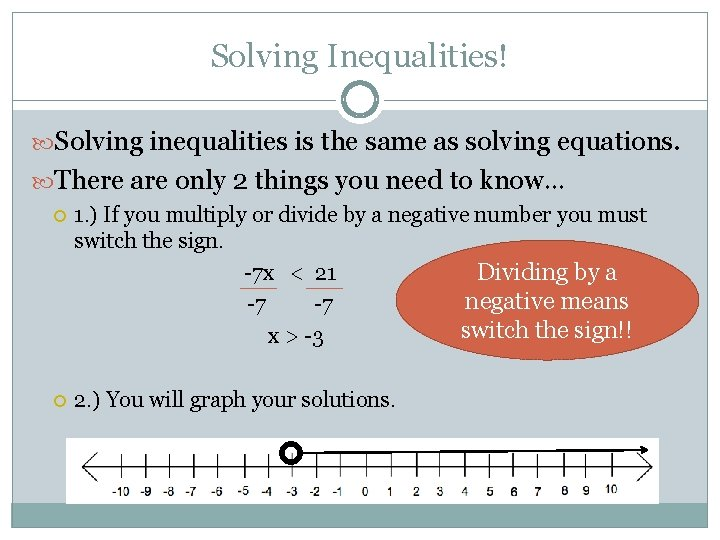Solving Inequalities! Solving inequalities is the same as solving equations. There are only 2 things you need to know… 1. ) If you multiply or divide by a negative number you must switch the sign. -7 x < 21 Dividing by a negative means -7 -7 switch the sign!! x > -3 2. ) You will graph your solutions.Special Case 1: Switching the Signs When solving inequalities, if you multiply or divide by a negative you must switch the signs. Switching the signs: Less than becomes Greater than < switches to Greater than becomes Less than > switches to Less than or equal to becomes Greater than or equal to ≤ switches to Greater than or equal to becomes Less than or equal to ≥ switches to > < ≥ ≤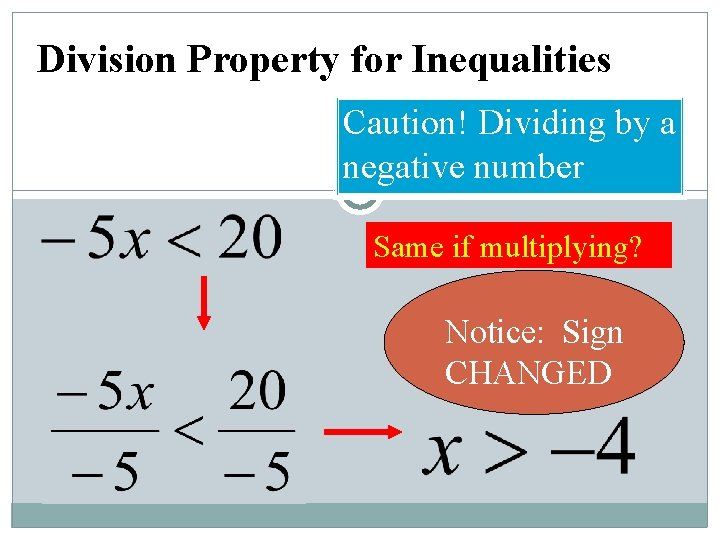Division Property for Inequalities Caution! Dividing by a negative number Same if multiplying? Notice: Sign CHANGEDMultiplication Property for Inequalities YES! -x > 2 5 (-5 )-x > 2(-5) 1 5 Caution! When you multiply by a negative number… …the sign CHANGES x < -10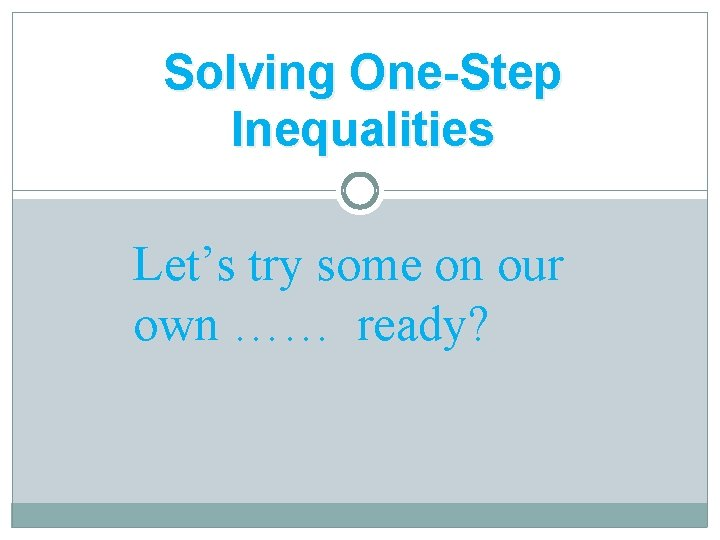Solving One-Step Inequalities Let’s try some on our own …… ready?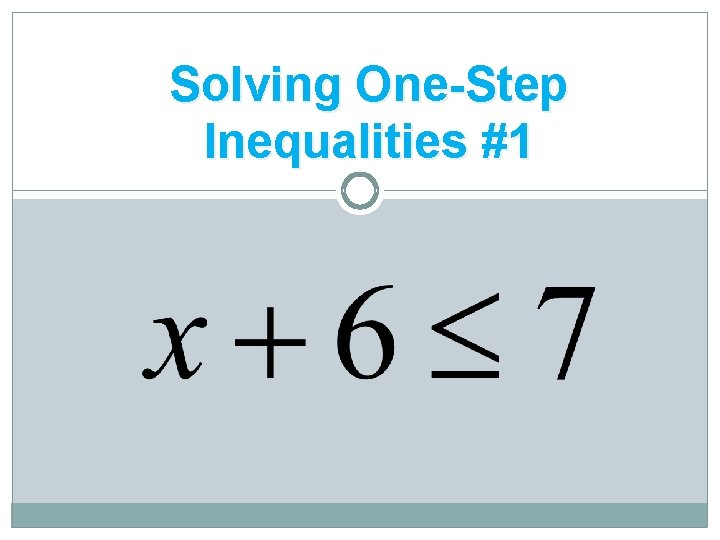Solving One-Step Inequalities #1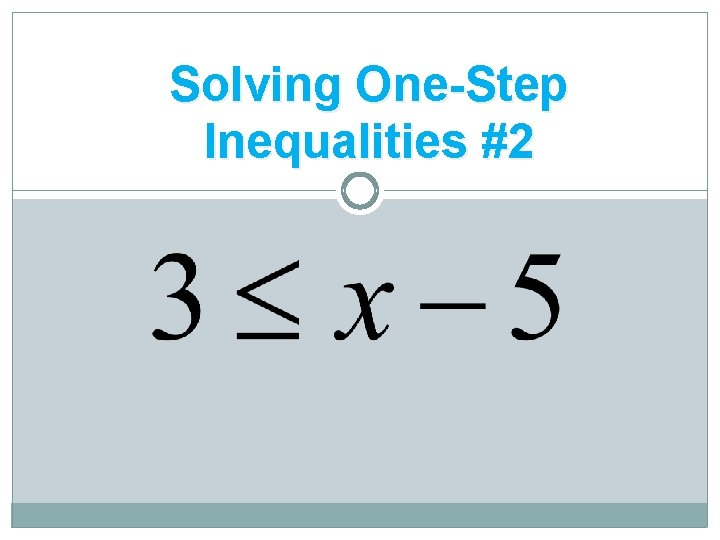Solving One-Step Inequalities #2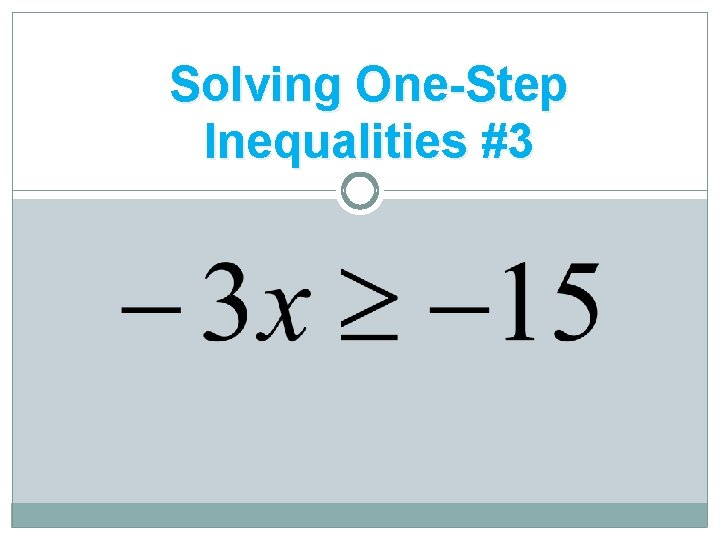Solving One-Step Inequalities #3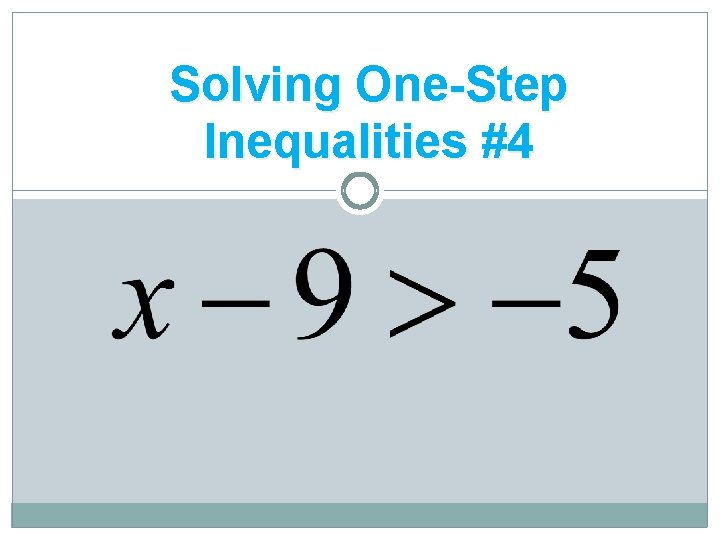Solving One-Step Inequalities #4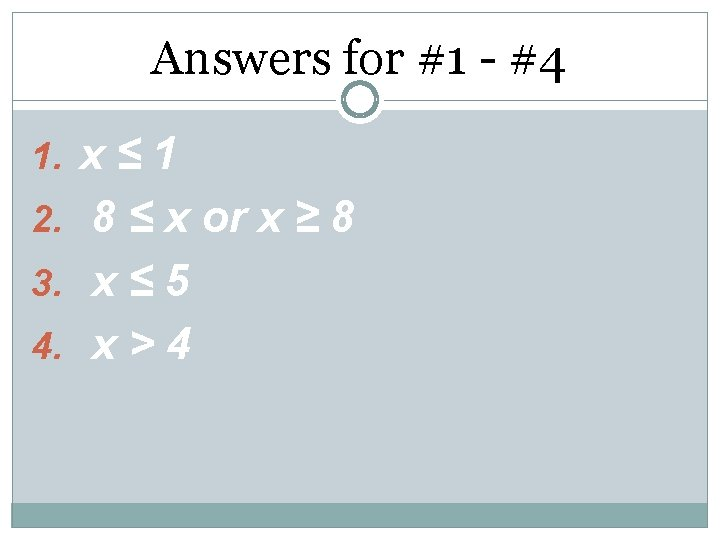Answers for #1 - #4 1 2. 8 ≤ x or x ≥ 8 3. x ≤ 5 4. x > 4 1. x ≤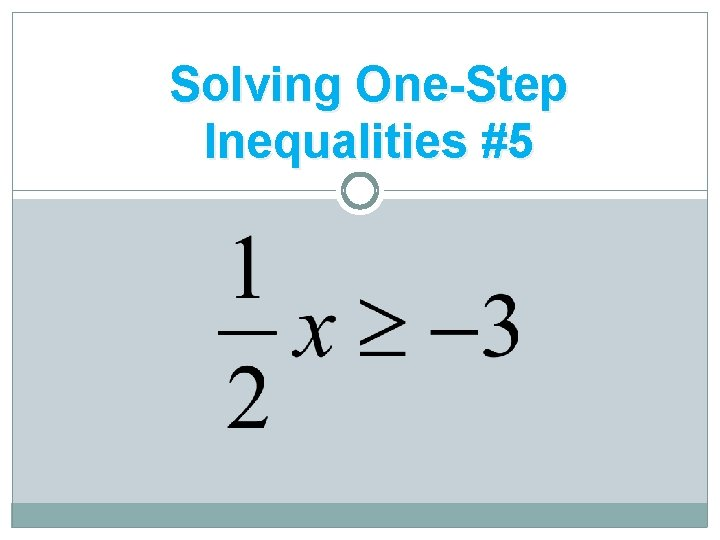Solving One-Step Inequalities #5Solving One-Step Inequalities #6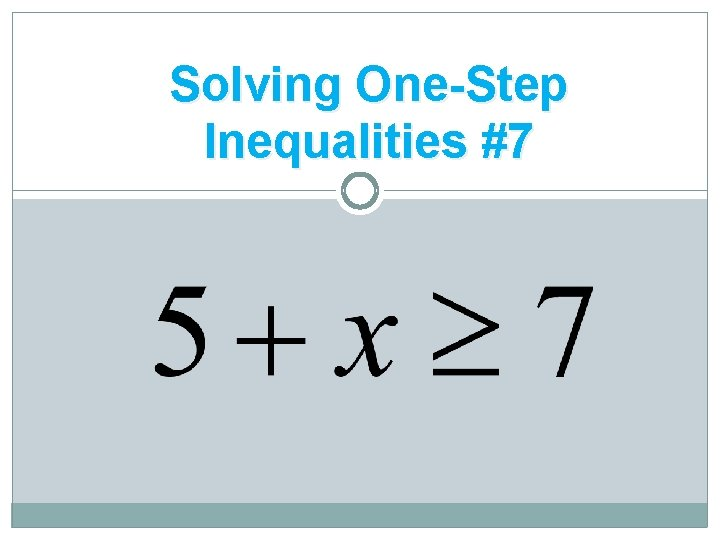Solving One-Step Inequalities #7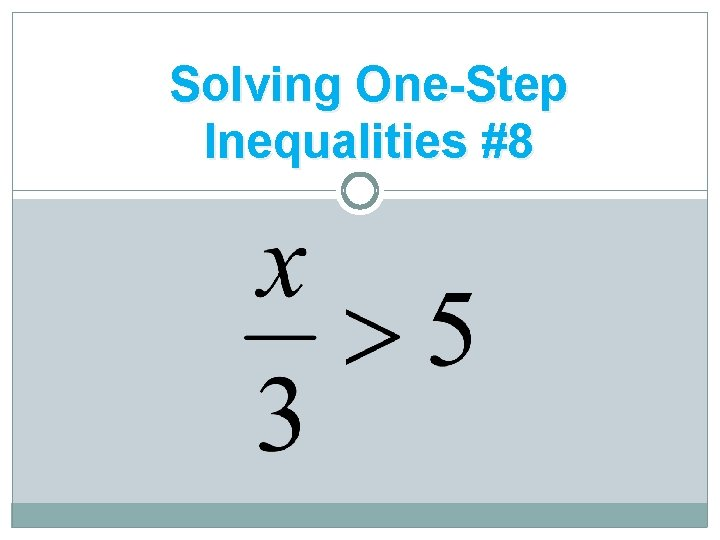Solving One-Step Inequalities #8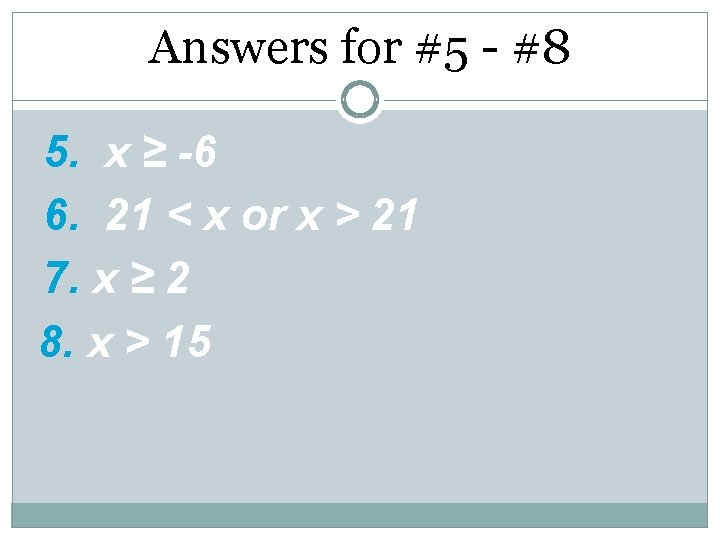Answers for #5 - #8 5. x ≥ -6 6. 21 < x or x > 21 7. x ≥ 2 8. x > 15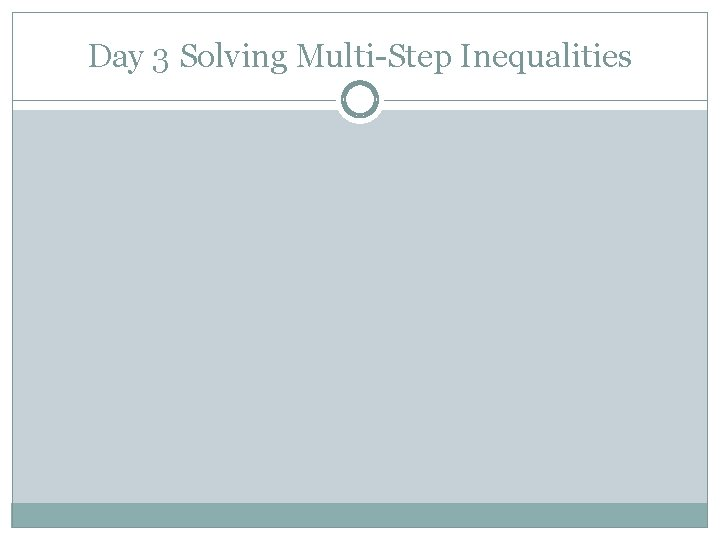Day 3 Solving Multi-Step Inequalities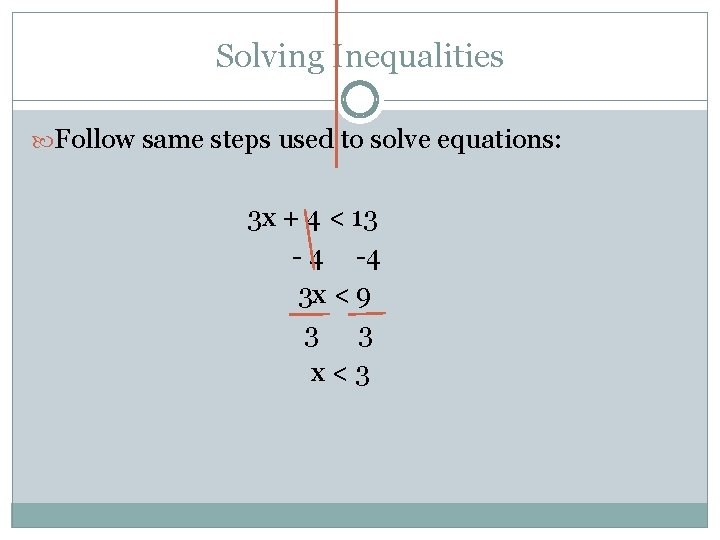Solving Inequalities Follow same steps used to solve equations: 3 x + 4 < 13 - 4 -4 3 x < 9 3 3 x<3Practice -2 x + 5 > 15 17 – 3 x < 41 14 > x + 4 x <7 -4 -5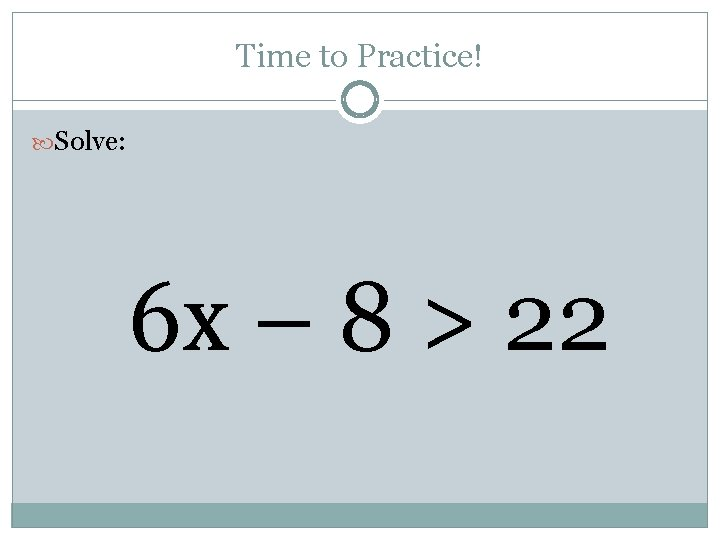Time to Practice! Solve: 6 x – 8 > 22Practice Problem 1 − 4 − 5 v < − 29Practice Problem 2 − 1 + 4 x ≤ 31Practice Problem 3 − 2 + r > − 1 9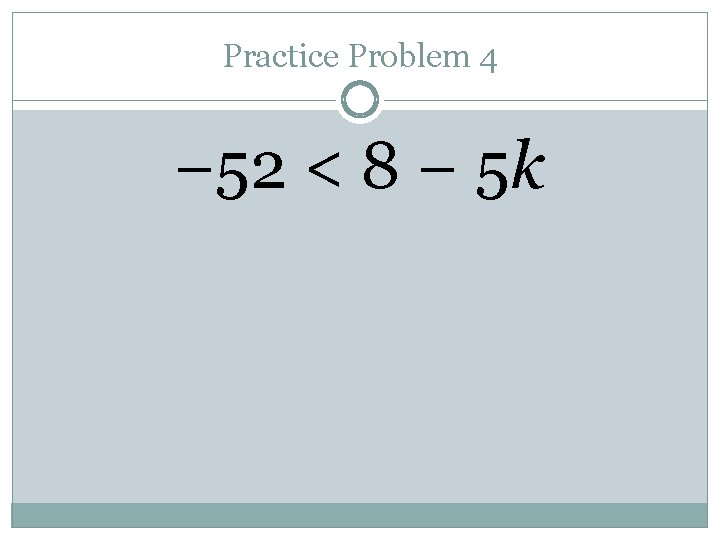Practice Problem 4 − 52 < 8 − 5 k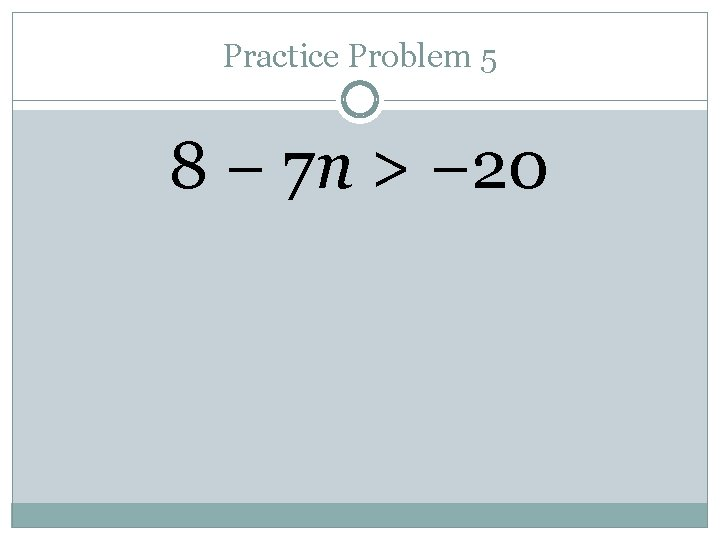Practice Problem 5 8 − 7 n > − 20Practice Problem 6 − 9 ≥ − 8 + v − 6